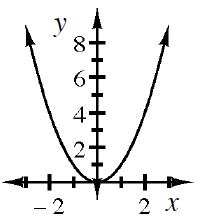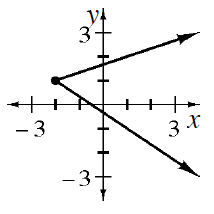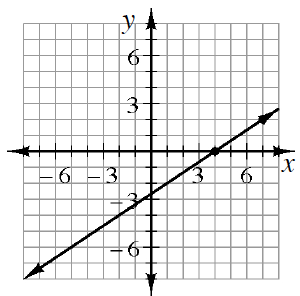### Home > INT2 > Chapter 4 > Lesson 4.1.1 > Problem4-10

4-10.

A relationship between inputs and outputs is a function if there is one and only one output for each input. Using this definition, decide if the relationships below are functions or not.

Are there any $x$-values on these graphs or in the table that have multiple $y$-values?

1.1.1.  $x$ $y$ $-8$ $-4$ $-1$ $-3$ $0$ $-2$ $1$ $-1$ $8$ $0$
1.